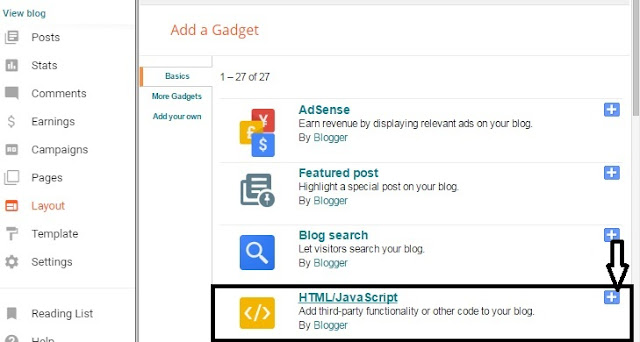# How To Add Canvas Clock Widget To Blogger /Blogspot

## How To Add Canvas Clock To  Blogger

• Select Html Java script
• A new page will open
• Copy the code from here which is given below and
• Past the code in the content section .
• And Click on  Save
• Now open your blog and watch it.Go>>Page>New Page >paste the code in page Html Section.

#### Copy the code from here to Add the Canvas Clock To blogger

<!DOCTYPE html>
<html>
<body>
<canvas id="canvas" width="300" height="300"
style="background-color:#FBFAFA">
</canvas>
<script>
var canvas = document.getElementById("canvas");
var ctx = canvas.getContext("2d");
var radius = canvas.height / 2;
setInterval(drawClock, 1000);
function drawClock() {
}
ctx.beginPath();
ctx.fillStyle = 'white';
ctx.fill();
ctx.stroke();
ctx.beginPath();
ctx.fillStyle = '#333';
ctx.fill();
}
var ang;
var num;
ctx.font = radius*0.15 + "px arial";
ctx.textBaseline="middle";
ctx.textAlign="center";
for(num = 1; num < 13; num++){
ang = num * Math.PI / 6;
ctx.rotate(ang);
ctx.rotate(-ang);
ctx.fillText(num.toString(), 0, 0);
ctx.rotate(ang);
ctx.rotate(-ang);
}
}
var now = new Date();
var hour = now.getHours();
var minute = now.getMinutes();
var second = now.getSeconds();
//hour
hour=hour%12;
hour=(hour*Math.PI/6)+
(minute*Math.PI/(6*60))+
(second*Math.PI/(360*60));
//minute
minute=(minute*Math.PI/30)+(second*Math.PI/(30*60));
// second
second=(second*Math.PI/30);
}
function drawHand(ctx, pos, length, width) {
ctx.beginPath();
ctx.lineWidth = width;
ctx.lineCap = "round";
ctx.moveTo(0,0);
ctx.rotate(pos);
ctx.lineTo(0, -length);
ctx.stroke();
ctx.rotate(-pos);
}
</script>
</body>
</html>

### Customization in Canvas Clock widget for blogger

You can change these setting in Canvas clock widget
Width = 300
Height = 300
Background Color = #FBFAFA

#### Final Words !

Thank you for following us if you face any problem in this post How To Add Canvas Clock Widget To  Blogger /Blogspot or another problem related with blogger .Then Contact us or comment us .We will solve your problem as soon as soon is possible. this is satisfied Website .From here you can get all hints tips and tricks for your blogger.
• Is this is tutorial is helpful ?
• Need to know your opinion